# How to Filter Data from One Sheet Based on Another Sheet in Excel?

We often filter data by some criteria in its own worksheet, but if we have two or more worksheets and want to filter data from sheet1 by the criteria in worksheet2 how can we do? If we can filter data by another worksheet, then in our daily work, it will be quite helpful when we have a summary list and filter data by some details by another worksheet. This article will show your two ways to do filter data by another worksheet, you can filter data by Advanced Filter function, or COUNTIF function.

Prepare two worksheets in one Excel file, and sheet1 records the summary of name & class & score, and sheet2 only records the name.

Sheet1: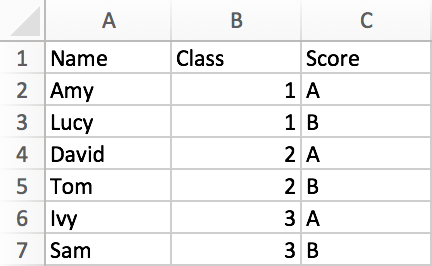Sheet2: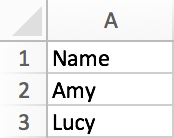If we only want to filter data in sheet1 by sheet2 column A, how can we do?

## Filter Data Based on Another Worksheet by Advanced Filter Function

Step 1: In tool bar, click on Data->Advanced. Advanced is located just next to Filter icon.Step 2: In Advanced Filter window, keep default selected option ‘Filter the list, in-place’, in List range, enter the range you want to do filter, in this case enter \$A\$1:\$A\$7. Don’t forget to add \$ before cell row and column number.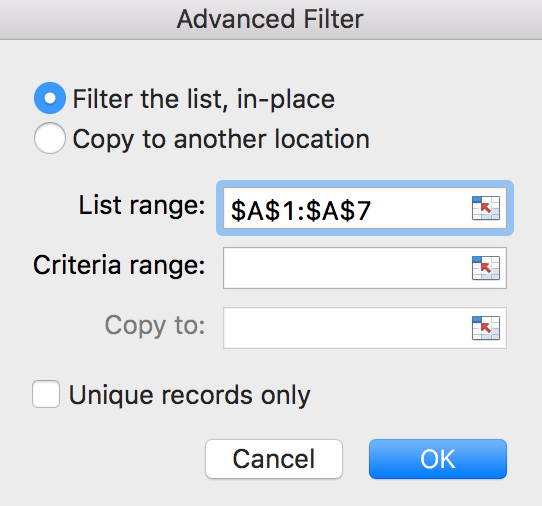Or you can directly click on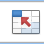button to select filter range by select cells on table directly.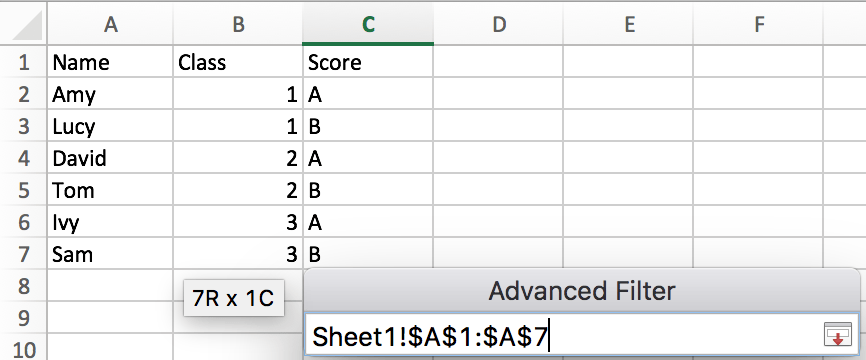Step 3: In Criteria range, clickbutton to select criteria range on sheet2.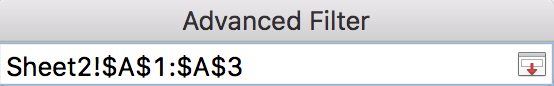Then we get criteria range on Advanced Filter, see below: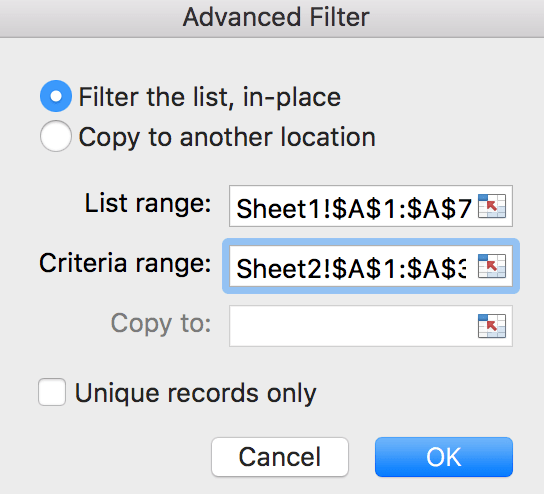Step 4: Click OK. Then we filter data based on shee2 column A successfully.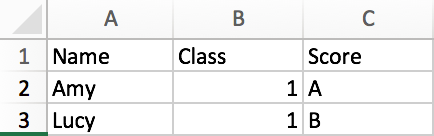## Filter Data Based on Another Worksheet by COUNTIF Function

Actually, we can use the COUNTIF function to calculate whether the data in sheet1 column A exists in sheet2 column A, and then use the filter function to filter the values that match the conditions according to sheet2 column A.

Step 1: In cell D2, enter the formula =COUNTIF(Sheet2!\$A\$1:\$A\$3,A2).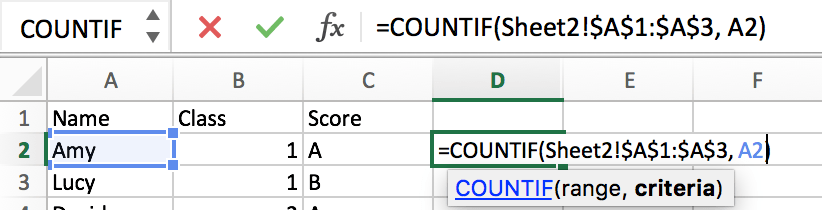Step 2: Press Enter. Then we get return value 1. Return value 1 means we can find A2 value in sheet2 range A1 to A3, and this value occurs only once.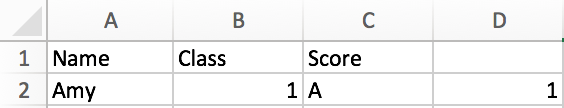Step 3: Copy the cell D2 with formula to other cells like below. Then we get all return values.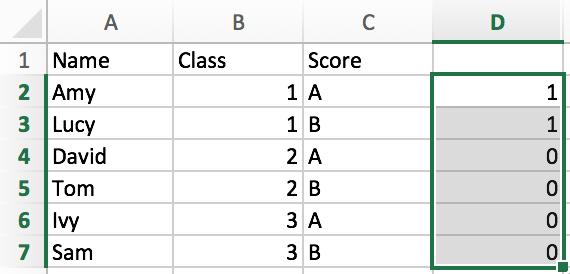Step 4: Do filter on column D. As we want to do filter by the criteria in sheet2 column A, so we only keep the rows which its D column value is 1. Select on D column in sheet1, click on Data->Filter, check on filter value 1, then click OK. Then we get the same result.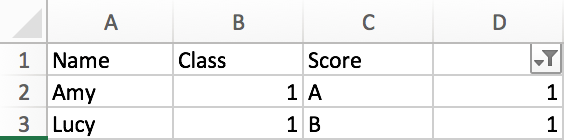### Related Functions

• Excel COUNTIF function
The Excel COUNTIF function will count the number of cells in a range that meet a given criteria. This function can be used to count the different kinds of cells with number, date, text values, blank, non-blanks, or containing specific characters.etc.= COUNTIF (range, criteria)…
Related Posts

If Cell Equals Certain Text String

IF function is frequently used in Excel worksheet to return you expect “true value” or “false value” based on the result of created logical test. If you want to see if cell equals a certain text string like “Win”, you ...

If Cell Contains Either Text1 or Text2

IF function is frequently used in Excel worksheet to return “true value” or “false value” based on the logical test result. If you want to see if cell contains certain substring1 like “abc” or substring2 like “def”, and returns true ...

If Cell Begins with One of Three Supplied Characters

If you want to test values to see if they begin with some given specific characters like “x”, ”y”, or “z”, you can create a formula with COUNTIF and SUM functions to return results. EXAMPLE You can see “TRUE” or ...

Average per Week by Formula in Excel

We usually apply AVERAGE function or relevant functions to return average directly in Excel worksheet. But in some situations, only applying average relevant functions cannot figure out our problem. Sometimes we can create a formula with functions and mathematical operation ...

How to Statistic Pass 4 Out of 5 Rounds in a Competition by Excel Functions

Suppose you are counting the numbers of participants who are the winners in a competition. And the passing line is provided as pass 4 out of 5 rounds of games. You need to know if participant pass the game or ...

How to Subtotal Values for Groups and Only Keep One Subtotal for A Group in Column

Sometimes we may meet the case that subtotal values for different groups and only record a subtotal value for one group in a column, for other cells in this group, keep them blank. Today we will introduce you how can ...

Running Count of Occurrence List

This post will guide you how to get running count of an occurrence in a list with formula in Excel 2013/2016 or Excel office 365. How to create a running count of certain values that appear in the given range ...

Count Non-Contiguous Range using COUNTIF

This post will guide you how to count a non-contiguous range with criteria in COUNTIF function in Excel 2013/2016 or Excel office 365. How do I build a formula for counting non-contiguous range in Excel using COUNTIF function in Excel. ...

How to Count Unique Numeric Values in a Range in Excel

This post will guide you how to count unique numeric values in the given range in Excel 2013/2016 or Excel office 365. How do I count the unique numeric values in a list of data with some duplicate values using ...

How to Count Unique Dates in Excel

This post will guide you how to count unique dates in a given range in Excel 2013/2016 or Excel office 365. How do I count the unique dates in a list of dates with some duplicate dates using a formula ...

Sidebar